Predicted Values

For a given set of covariates,(including the intercept term), theth quantile of the log response,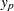, is given by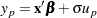if no offset variable has been specified, or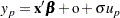for a given value o of an offset variable, where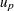is theth quantile of the baseline distribution. The estimated quantile is computed by replacing the unknown parameters with their estimates, including any shape parameters on which the baseline distribution might depend. The estimated quantile of the original response is obtained by taking the exponential of the estimated log quantile unless the NOLOG option is specified in the preceding MODEL statement.

The following table shows howis computed from the baseline distribution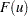:

DistributionExponential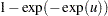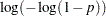Generalized Gamma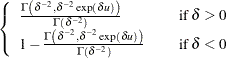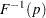Logistic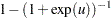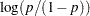Log-logisticLognormal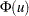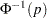NormalWeibullFor the generalized gamma distribution,is computed numerically.

The standard errors of the quantile estimates are computed using the estimated covariance matrix of the parameter estimates and a Taylor series expansion of the quantile estimate. The standard error is computed as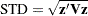where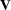is the estimated covariance matrix of the parameter vector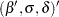, andis the vector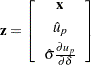where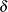is the vector of the shape parameters. Unless the NOLOG option is specified, this standard error estimate is converted into a standard error estimate for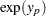as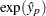STD. It might be more desirable to compute confidence limits for the log response and convert them back to the original response variable than to use the standard error estimates fordirectly. See Example 50.1 for a 90% confidence interval of the response constructed by exponentiating a confidence interval for the log response.

The variable CDF is computed as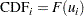where the residual is defined by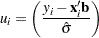andis the baseline cumulative distribution function. If the data are interval-censored, then the cumulative distribution function,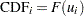, is evaluated at the lower interval endpoint.